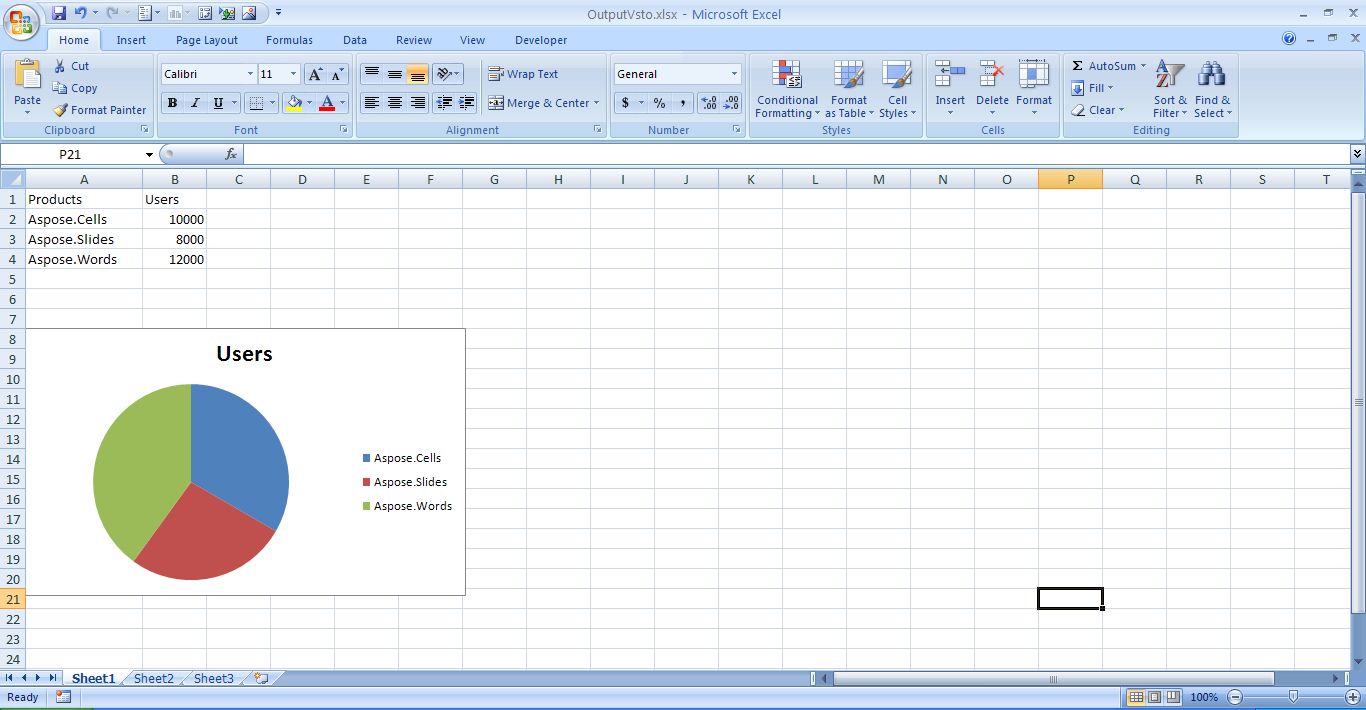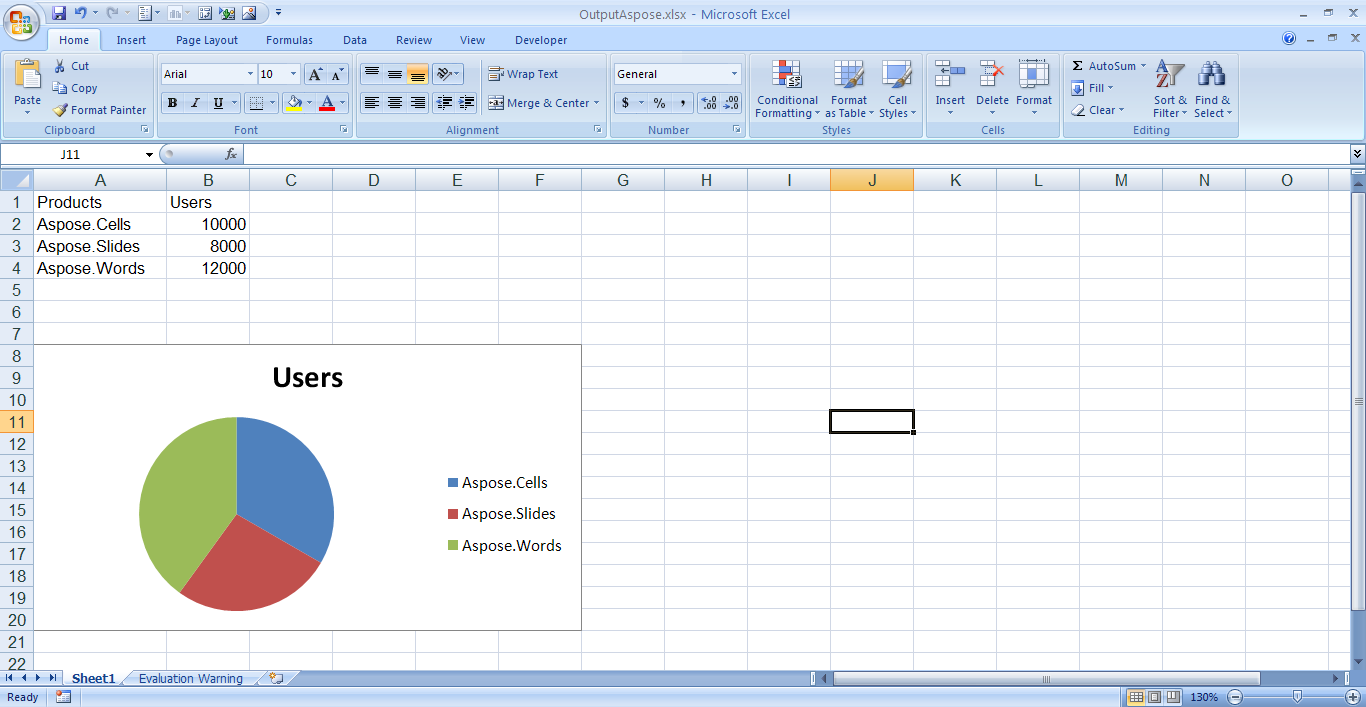# Create a Pie Chart

Contents
[ ]

## Creating a Pie Chart

This article compares how to create a pie chart using Office Automation and VSTO to using Aspose.Cells for .NET. The steps for creating a pie chart are:

1. Creating a workbook and worksheet.
3. Referencing productsChart.
4. Adding a pie chart, defining the data range and chart title.

The code samples in this article show how to add a pie chart with VSTO, using either C# or Visual Basic, compared to creating one with Aspose.Cells, again using either C# or Visual Basic.

### Creating a Pie Chart with VSTO

The code samples that follow show how to add a pie chart to a spreadsheet using VSTO.

C#

`````` void PieChart()

{

//Access a Vsto Worksheet

Microsoft.Office.Tools.Excel.Worksheet sheet = this;

//Add sample data for pie chart

sheet.Cells[1, 1] = "Products";

sheet.Cells[1, 2] = "Users";

//Add data from A2 till B4

sheet.Cells[2, 1] = "Aspose.Cells";

sheet.Cells[2, 2] = 10000;

sheet.Cells[3, 1] = "Aspose.Slides";

sheet.Cells[3, 2] = 8000;

sheet.Cells[4, 1] = "Aspose.Words";

sheet.Cells[4, 2] = 12000;

//Chart reference

Microsoft.Office.Tools.Excel.Chart productsChart;

productsChart = sheet.Controls.AddChart(0, 105, 330, 200, "ProductUsers");

productsChart.ChartType = Microsoft.Office.Interop.Excel.XlChartType.xlPie;

//Set chart title

productsChart.HasTitle = true;

productsChart.ChartTitle.Text = "Users";

//Gets the cells that define the data to be charted.

Microsoft.Office.Interop.Excel.Range chartRange = sheet.get_Range("A2", "B4");

productsChart.SetSourceData(chartRange, missing);

//Access the Active workbook from Vsto sheet

Microsoft.Office.Interop.Excel.Workbook workbook= sheet.Application.ActiveWorkbook;

//Save the copy of workbook as OutputVsto.xlsx

}
``````

A pie chart created with VSTO### Creating a Pie Chart with Aspose.Cells for .NET

The code samples that follow show how to add a pie chart to a spreadsheet using Aspose.Cells.

C#

`````` private void PieChart()

{

//Create Aspose.Cells Workbook

Workbook workbook = new Workbook();

//Access Aspose.Cells Worksheet

Worksheet sheet = workbook.Worksheets;

//Add sample data for pie chart

sheet.Cells["A1"].PutValue("Products");

sheet.Cells["B1"].PutValue("Users");

//Add data from A2 till B4

sheet.Cells["A2"].PutValue("Aspose.Cells");

sheet.Cells["B2"].PutValue(10000);

sheet.Cells["A3"].PutValue("Aspose.Slides");

sheet.Cells["B3"].PutValue(8000);

sheet.Cells["A4"].PutValue("Aspose.Words");

sheet.Cells["B4"].PutValue(12000);

//Chart reference

Chart productsChart;

int chartIdx = sheet.Charts.Add(ChartType.Pie, 7, 0, 20, 6);

productsChart = sheet.Charts[chartIdx];

//Gets the cells that define the data to be charted

Series nSeries = productsChart.NSeries[seriesIdx];

nSeries.XValues = "=Sheet1!\$A\$2:\$A\$4";

//Set chart title

productsChart.Title.Text = "Users";

//Autofit first column

sheet.AutoFitColumn(0);

//Save the copy of workbook as OutputAspose.xlsx